Science Journal of Applied Mathematics and Statistics
Volume 5, Issue 1, February 2017, Pages: 24-30

A Trinomial Probability Model for Occurrences of Stock Price Change: Evidence from Dhaka Stock Exchange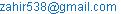(Md. Z. Islam)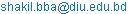(S. Ahmad)

Md. Zahirul Islam, Shakil Ahmad. A Trinomial Probability Model for Occurrences of Stock Price Change: Evidence from Dhaka Stock Exchange. Science Journal of Applied Mathematics and Statistics. Vol. 5, No. 1, 2017, pp. 24-30. doi: 10.11648/j.sjams.20170501.14

Received: December 8, 2016; Accepted: December 19, 2016; Published: January 18, 2017

Abstract: This paper is concerned with modeling the occurrences of stock price uncertainty of Dhaka Stock Exchange. Daily closing prices of three different banks are selected for analysis. This report focuses on the overall condition of the stock market to find out the amount of probability of uncertainty of occurrences by analytically chosen model to the financial data of Banking Sector (leading three Banks of Bangladesh: AB Bank, City Bank and National Bank). Various popular variability-forecasting models with techniques of measuring and evaluating performance of forecasting were reviewed. In this research, a trinomial probability distribution model is fitted considering the outcome (closing price) of a stock (per day) such as low, unchanged and high for the quoted three banks. Maximum likelihood estimations are derived for estimating the parameters of the model. To check the model acceptability chi-square goodness-of-fit test is conducted. It is found that the probability of occurrences of unchanged price for AB bank is low (0.014). On the other hand the probability of occurrence of high and low price are high (0.478 and 0.508) and these probabilities are almost same for the other banks (City and National bank).

Keywords: Trinomial Probability Model, Dhaka Stock Exchange, Time Series Analysis, Stock Price and Stock Market

Contents

1. Introduction

Development such as financial development, economic development etc. of a country depends on making policy of this country and interest in financial markets and the possibility to forecast their course is connected to the growing recognition among economists, financial analysts and policy makers because of the increasing impact of financial variables on the macro economy. Stock price variability has been the focus of regulators, academic researchers and practitioners concern. Stock markets tend to be very efficient in the allocation of capital to its highest value users. These markets also help increase savings and investment, which are essential for economic development. An equity market, by allowing diversification across a variety of assets, helps to reduce the risk of the investors must bear, thus reducing the cost of capital, which in turn spurs investment and economic development. For example, in a stock market, which is informatively inefficient, investors face difficulty in choosing the optimal investment as information on corporate performance is slow or less available. The globalization and integration of these markets has created enormous opportunities for domestic and international investors to diversify their portfolios across the globe. As a result, rigorous empirical studies examining the efficiency and other characteristics of these markets would be of great benefit to investors and policy makers at home and abroad. According to Kim, exchange rate is one of the major determinants of business Profitability and equity prices.

Rahman and Uddin explored the relationship between stock prices and exchange rates by using Johansen cointegration and Granger causality test in three emerging South Asian countries - Bangladesh, India and Pakistan and found neither cointegrating relationship nor any causal relationship between stock prices and exchange rates in the countries. However, Alam & Uddin studied the linear relationship between share price and monetary policy, particularly between share price and changes in interest rate on DSE. Evidences from his study showed that monetary policy has significant negative relationship with share price.

Our main interest is to determine the variability of a financial data set and the measure of tradeoff between return and risk for allocating assets and is closely related to portfolio return fraction which is central to risk management measure like VaR (Value at Risk). Since the daily closing price change is not unique or probability associated, our main intention is to build up a probability model. If we consider three states as low, unchanged and high of the occurrences of daily closing price as decrease, unchanged and increase respectively then may easily fit the trinomial distribution model in order to assess the amount of uncertainty of the stock price. In the successive chapters, we will go on establishing the trinomial distribution model and observe its overall performance on price change. We will find the probabilities of how many days the closing price decrease, how many days it will remain unchanged and how many days it will increase in the future. As a result, it will be a guideline for the new investors who want to achieve good return from his investment.

Objectives of the study: In this paper our main objectives are:

To develop a probability model on stock market data.

To explain the nature of trinomial distribution model.

To test the goodness of fit of the model.

To see the development of the company.

2. Concept on Stock Market

For any country, share market is a pillar of economics. For economic development of any country, the importance of share market is very necessary. By investing in share market, the shareholders can be a partner in the development of the country. A share or stock is a document issued by a company, which entitles its holder to be the owner of the company. By owning a share, you can earn a portion and selling it you can get capital gain. So your return is the dividend plus the capital gain. However, you also run a risk of making a capital loss if you have sold the share at a price below your buying price.

A stock market is private or public market for the trading of company stock and derivatives of company stock at an agreed price; these are securities listed on a stock exchange as well as those only traded privately. The face value of a share at the end of the each trading day is termed as closing price. Most stocks are traded on exchange. As a result, Closing price changes every day for the most of the stock either low or high, or it may remain unchanged.

2.1. Causes of Price Change

Stock price changes everyday due to market forces. By this, we mean that share price changes because of supply and demand. Any single time, if more people want to buy a stock (demand) than sell it (supply), then the price moves up. Conversely if more people want to sell a stock than buy it, which is a greater supply than demand, then the price falls.

2.2. Data Description

Several indices are available for Bangladesh Stock Market. The index includes all share price index, DSE 20 index and general index. The index used in this study is the DSE 20 index. Here we consider daily data. Our sample consists of daily closing price from three banks: AB Bank, City Bank and National Bank in the Dhaka Stock Exchange.

2.3. Method of Data Collection

We can collect data by different types of methods in different places, where which is applicable. In this study for collecting data, we use non-probability sampling method.

2.4. Data Collection

We have collected data from the library of Dhaka Stock Exchange from where we collect daily stock market data, which is financial time series data. We have collected secondary data on share market of 2006.

2.5. Nature of Data

The data is incomplete. In the data set, there are some missing observations. For removing the brake and for continuity we use the mean substitution technique to estimate the missing values.

2.6. Missing Value Estimation

Estimation of missing values for time series data is quite different. Missing data occur in a wide array of application domains for a variety of reasons. In share market one company may not able to trade in all working day and may occur a missing. There are many reasons for such missing occurrence such as various political crises, company’s miss-management system, rough weather and other economic factor, which may play vital in such inability of a company to participate in share market. (See Marlin, B. M. 2008).

In this study our main task is to measuring and forecasting the daily price change of stock and observing their switching in different specified (state-1, low price than previous day, state-2, medium price than previous day, state-3, high price than previous day) for achieving such defined objective we face a serious problem for the presence of missing data. In these notes, we give an overview of estimation of missing data in time series data.

2.7. Missing Imputation of Dhaka Stock Market

It is very important to estimate the missing values in these situations. We pursue the very simple calculative procedure to find out the missing value. One of these procedures is that calculates the average value for missing one between two existing values. Others are: Mean substitution, Single exponential method, Interpolation, Extrapolation and Mean/Median of nearby points

2.8. Data Coding

After estimating the missing observations, we code each observation to fit with the target distribution model. We transform the observations into three categories such as

1     if the closing price of a stock decreases than the previous day which is termed as "low",

2     if it remains the same which is termed as "unchanged" and

3     if it increases than the previous day which is termed as "high".

After coding the each observation, we will find the frequency of the each category for each of the three banks.

Randomness Test of the Data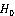: The sequence is random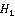: The sequence follows trend

The test statistic is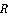= Total no. of runs

For small sample (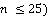the P-values are as follows:

Table 1. For small sample (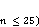the P-values are as follows.

 Alternative Hypothesis P-value (i) A tendency to cluster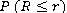(ii) A tendency to mix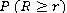(iii) Trend Small of above 2

Table 2. For small sample (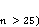the P-values are as follows.

 Alternative Hypothesis P-value (i) A tendency to cluster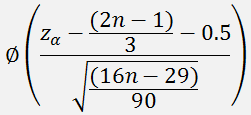(ii) A tendency to mix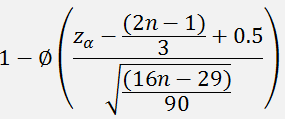(iii) Trend Small of above 2 replacing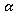by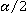2.9. Goodness-of-Fit Test

The compatibility of a set of observed sample values with a distribution can be checked by a good-of-fit type of test. In order to apply the chi-square test at our data, the sample data must first be grouped according to categories of low, unchanged and high in order to form a frequency distribution.

Assume that the 30 observations have been grouped into k mutually exclusive categories, and denote by fi and ei the observed and expected frequencies, respectively, for the class i= 1, 2,…, k. The decision regarding fit is to be based on the deviations fi-ei. The sum of these k deviations is zero except for rounding. The test criterion suggested by Pearson (1900) is the random variable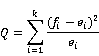A large value of Q would reflect an incompatibility between the observed and expected relative frequencies, and therefore the null hypothesis on which ei were calculated should be rejected for Q large.

3. Descriptive Statistics

The descriptive statistics for the data of three banks collected from the Dhaka Stock Market on daily closing price (in 2006) is presented in the following table:

Table 3. Comparison of Descriptive Statistics of three banks on closing price.

 Descriptive Statistics AB Bank City Bank National Bank Mean 545.95 580.39 720.24 Standard Error 12.23 4.68 3.51 Median 412.97 571.25 737.5 Mode 363 564.5 749.25 Standard Deviation 233.83 89.41 67.09 Sample Variance 54678.99 7994.55 4500.89 Kurtosis -1.49 0.23 0.263 Skewness 0.45 -0.24 -0.95

From the above table we see that the standard deviation or sample variance of daily closing price is smaller for National bank than the other two. Therefore, the price variation as well as the risk is low for National Bank than the other two Banks.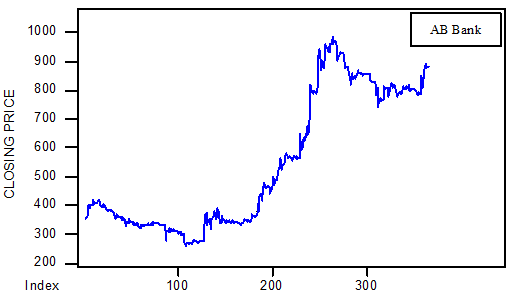Figure 1. The time series plot for the data of AB Bank.

The above is a plot of the closing price change of AB bank is random. Visual inspection of this graph suggest that initially price of stock is decreasing up to 100 days, but for 100th to 275 days it is absolutely increasing. After 275th day, again it is decreasing with obvious variations.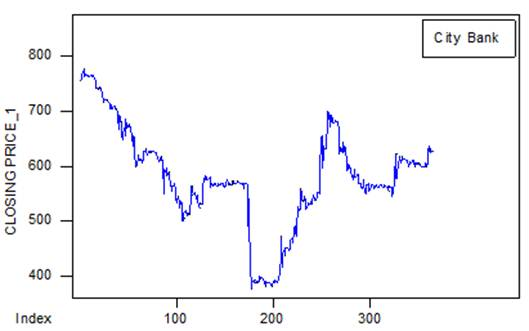Figure 2. The time series plot for the data of The City bank.

The above is a plot of the closing price change of City bank is random. Visual inspection of this graph suggest that initially it is decreasing up to 100 days, but for 100 to 165 days it is almost steady with a little bit fluctuation. After 165th day, suddenly price fall, it is increasing up to 260 days while price fall at the end of the obvious variations.

The above is a plot of the closing price change of National bank is random. Visual inspection of this graph suggest that initially it is decreasing up to 110 days, but for 100th to 160th days it is mare volatile with suddenly increase and fall of the price. After 160th day, it is increasing with obvious variations.

As we develop the probability model, it needs to consider whatever the data is random or not. In addition, it is not necessary to consider the time effect, seasonal variation or trend.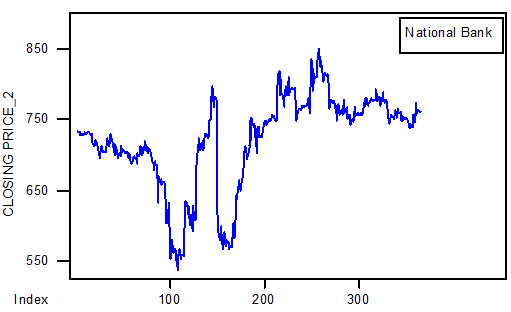Figure 3. The time series plot for the data of National bank.

3.1. Bar Chart

The charts are constructed based on the data obtained from the Dhaka stock exchange on daily closing price of three banks.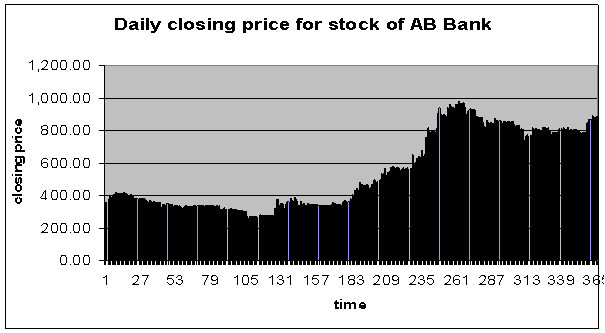Figure 4. Bar chart for the data of AB Bank.

From the above chart, we see that there is no regular variation in the data set. The price is slightly decreasing up to 105 days with initial 10-day’s increase. Then gradually price is increasing up to 265th day. After then the price is almost same.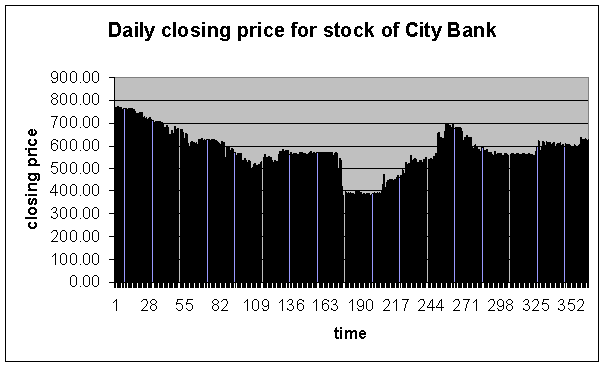Figure 5. Bar chart for the data of City Bank.

From the above chart, we see that there is no regular variation in the data set. The price is absolutely decreasing up to 120 days. Then the price is almost same from 120th day to 172th day with a sudden fall after 172th day. Then the price is again almost same from 175th day to 202th day. Then gradually price is increasing up to 267th day. After then the price is almost same with initial little bit fall.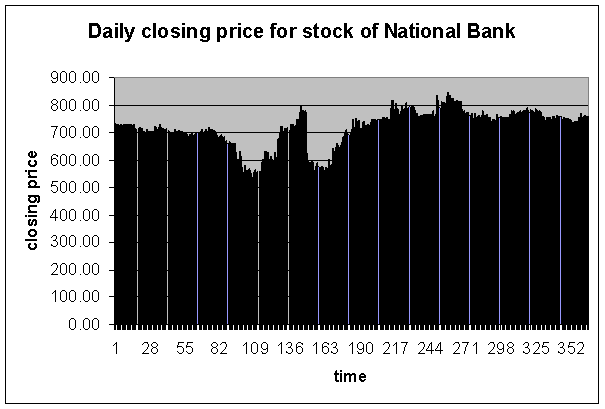Figure 6. Bar chart for the data of National Bank.

From the above chart, we see that there is no regular variation in the data set. The price is almost same over the year except the variation within the days of 80 to 190.

3.2. Probability Modeling

In this part, our main objective is to develop a probability model for the data of daily closing price collected from the Dhaka Stock Market. We find the maximum likelihood estimate of the unknown parameters of fitted model. Finally, we perform the goodness of fit test in order to assess the efficiency of fitness of the model to the data.

3.3. Visual View of the Occurrence of Daily Closing Price

We transform the observations into three categories such as 1= if the closing price of a stock decreases than the previous day which is termed as "low", 2= if it remains the same which is termed as "unchanged" and 3= if it increases than the previous day which is termed as "high".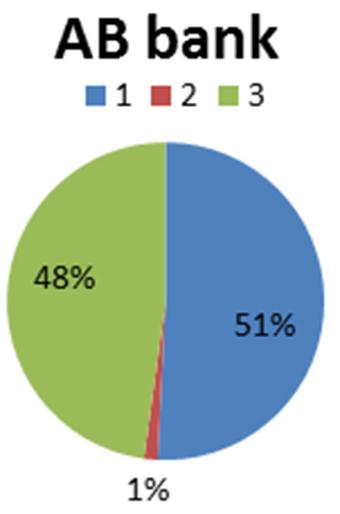Figure 7. Pie chart for AB Bank Ltd.

From the above chart, we see that the occurrence of "low" closing price is 51%, the occurrence of "unchanged" closing price is 1% and that of "high" closing price is 48%.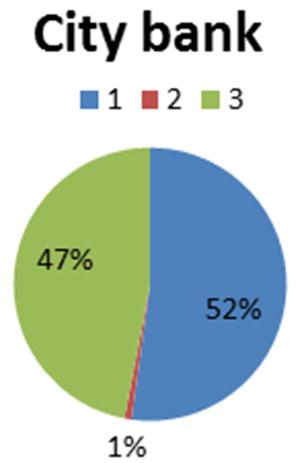Figure 8. Pie chart for City Bank Ltd.

From the above chart, we see that the occurrence of "low" closing price is 52%, the occurrence of "unchanged" closing price is 1% and that of "high" closing price is 47%.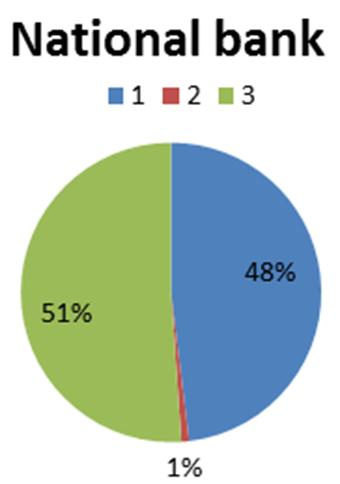Figure 9. Pie chart for National Bank Ltd.

From the above chart, we see that the occurrence of "low" closing price is 48%, the occurrence of "unchanged" closing price is 1% and that of "high" closing price is 51%.

3.4. Development of the Model

Suppose that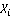denote the successive daily changes of the price of a given stock are assumed to be independent and identically distributed random variables with probability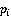. For the data of size n it is assumed that each observation may occur in three states such as 1= the closing price of a stock decreases than the previous day, 2= it remains unchanged and 3= it increases than the previous day. Then we can define that Xi follow the trinomial distribution with probability mass function: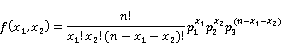Where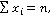and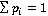.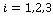and Mean=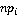and variance=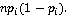3.5. Parameter Estimation

According to a paper of "Maximum Likelihood Estimation" by Chris Williams, School of Informatics, University of Edinburgh, the maximum likelihood estimate of the probability of trinomial distribution model,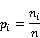3.6. Probability Determination

Table 4. Probability Distribution for One month period.

 Category One month period low unchanged probability 13 2 0.032 14 1 0.008 15 0 0.093 16 1 0.038 17 2 0.005

Here we have determined the probability for one, two and three month’s sample. In a sample of 30 days, the probability of occurrence that the price of a stock will be low any 13 days and remain unchanged any day is 0.032. It is also come to know that the probability decreases when the number of occurrence of unchanged price increases.

Table 5. Probability Distribution for Two months period.

 Category Two month period low unchanged probability 28 2 0.011 29 1 0.012 30 0 0.006 31 1 0.017 32 2 0.019

For two-month period, the probability is comparatively low. In case of a sample of 60 days a dramatically change is observed for unchanged price. For one-month data, the probability decreases when the number of occurrence of unchanged price increases but for a sample of 60 days observations, the probability decreases when the number of occurrence of unchanged price decreases.

Table 6. Probability Distribution for Three months period.

 Category Three month period low unchanged probability 43 2 0.005 44 1 0.004 45 0 0.001 46 1 0.005 47 2 0.010

For three-month period, the probability is lower than one or two month period sample. For a sample of 90 days observations, the probability decreases when the number of occurrence of unchanged price decreases.

4. Discussion

It can be extended for one-year data for the three banks as follows:

For the AB Bank:

Table 7. Frequency distribution for AB Bank (1-year data).

 AB BANK Ltd. Category Frequency,Probability,low 185 0.508242 Unchanged 5 0.013736 high 174 0.478022 total=n 364 1

Using the above data of the frequency distribution, we see that,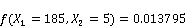This implies that for a given year, the probability of occurrence that the price of the stock will increase any 185 days than the previous day and the price of the stock will remain unchanged for any 5 days is 0.013795.

For the City Bank: For The City Bank, the frequency distribution of the category becomes:

Table 8. Frequency distribution for The City Bank.

 The CITY BANK Ltd. Category Frequency,Probability,low 190 0.521978 Unchanged 3 0.008242 high 171 0.46978 total=n 364 1

Using the above data of the frequency distribution, we see that,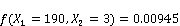.

Which implies that for a given year, the probability of occurrence of the price of the stock will decrease any 190 days than the previous day and the price of the stock will remain unchanged for any 3 days is 0.00945.

For National Bank:

For The National Bank Limited the frequency table of the states becomes

Table 9. Frequency distribution for National Bank.

 NATIONAL BANK Ltd. Category Frequency,Probability,low 175 0.480769 Unchanged 3 0.008242 high 186 0.510989 total=n 364 1

Using the above data of the frequency distribution, we see that,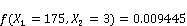Which implies that for a given year, the probability of occurrence of the price of the stock of National Bank will decrease any 175 days than the previous day and the price of the stock will remain unchanged for any 3 days is 0.013795.

Goodness of Fit Test: Suppose the null hypothesis is that, the data follow the trinomial distribution model. Since the data are grouped and the hypothesized null distribution is discrete, the chi-square goodness of fit test is appropriate. However, no parameters are specified so they must be estimated from the data in order to carry out the test.

The trinomial distribution is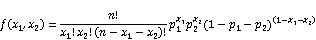For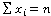. The maximum likelihood estimate ofis the proportion of each category in the sample as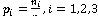.

Here

Table 10. Frequency Distribution for AB BANK.

 AB BANK Ltd. Category Frequency,Probability,low 185 0.508242 Unchanged 5 0.013736 high 174 0.478022 total=n 364 1

P1= n1/n= 185/364= 0.508242; P2= n2/n= 5/364=.013736; P2= (1- P1- P2) = 0.478022.

We use these value in f(x) to estimate the probabilities as θi to compute ei=720i. Notice that the final θ is not for exactly 21 low and 1 unchanged but for 21 or more and 1 or more unchanged; this is necessary to make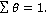The result is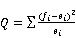= 25.40

With 17 degrees of freedom; we start with k-1= 18 degrees of freedom and lose one for estimating θ. The tabulated value for the chi-square distribution with 17 degrees of freedom is 27.587 at 5% level of significance. The value of the test statistic is less than the critical value, so we cannot reject the null hypothesis at 5% level of significance.

5. Summary and Conclusions

The mean of the closing price for AB bank is 545.95, which is smaller than that of City bank (580.39) and National bank (720.24) though the IPO face values are same. From this, we can conclude that the return from the National bank is high as the average is high which means that more and more price increases.

The standard deviation is the smallest for the closing price of National bank is 67.09 where it is 89.41 for the City bank and 233.83 for AB bank. Therefore, we can say the risk is minimum for National bank than City bank and AB bank.

From the visual inspection of the time series plots and bar charts, we observe that the variability is less in case of National bank than City bank and AB bank. Therefore, the investors can employ their investment buying the stock of the National bank without large amount of fear for better return.

As the chi-square goodness-of-fit test gives evidence of accepting the null hypothesis of trinomial distribution, which fits well to the data, one can easily use this distribution to find out the probability of uncertainty in the stock market. As a result, this paper will be a good guideline for the investors and practitioners.

References

1. Gibbons, J. D. and Chakraborti, S. (1992): Nonparametric Statistical Inference, 5th edition, Marcel Dekker, Inc.
2. Marcell Hogg, R. V. and Craig, A. T. (2002): Introduction to Mathematical Statistics, 5th edition, Pearson Education (Singapore) Pte Ltd.
3. Roy, M. K. (2004): Probability and Probability Distribution, Romax Publication, Chittagong, Bangladesh.
4. Mood, A. M., F. A. Graybill and D. C. Boes (1974): Introduction to the Theory of Statistics, 3rdedition, Tata McGraw-Hill.
5. Dhaka Stock Exchange Limited, Dhaka, Bangladesh.
6. An Appraisal of Hospital Based Blood Pressure Control in Port Harcourt, Nigeria.
7. "The Multinomial Distribution STAT 504". onlinecourses. science. psu. edu. Retrieved 2016-09-11.
8. Evans, Morton; Hastings, Nicholas; Peacock, Brian (2000). Statistical Distributions, 3rd Edition, New York: Willey. pp. 134–136. ISBN 0-471-37124-6.
9. Kim, K (2003) "Dollar Exchange Rate and Stock Price: Evidence from Multivariate Cointegration and Error Correction Model." Review of Financial Economics, 12, 301-313.
10. Rahman, M. L., & Uddin, J. (2009). "Dynamic Relationship between Stock Prices and Exchange Rates: Evidence from Three South Asian Countries", International Business Research, 2 (2), 167-174.
11. Alam, M., & Uddin, G. S. (2009). "Relationship between Interest and Stock Prices: Empirical Evidences from Developed and Developing Countries". International Journal of Business and Management, 4 (3), 43-51.
12.    Neri, S. (2004). Monetary Policy and Stock Price Theory & Evidence. Journal of Economic Literature 32, 7-47.

 Contents 1. 2. 2.1. 2.2. 2.3. 2.4. 2.5. 2.6. 2.7. 2.8. 2.9. 3. 3.1. 3.2. 3.3. 3.4. 3.5. 3.6. 4. 5.
Article ToolsAbstractPDF(344K)